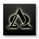จำนวนเข้าชม 6611
Squeeze Momentum Indicator - Optimized

All Updates from Version 3 + Squeeze Optimized further.
// Heikin Ashi Optimization Applied

study(shorttitle = "UCS_SQZ_Opt", title="UCS_Squeeze Momentum - Optimized", overlay=false)

length = input(20, title="Squeeze Length")
multBB = input(2,title="BB MultFactor")
multKC = input(1.5, title="KC MultFactor")
smooth = input(20, title = "Momentum Smoothing")

usebbr = input(true, title = "Use Bollinger Band Ratio Instead of Momentum", type = bool)
useHAC = input(true, title = "Heikin Ashi Optimization", type=bool)

// Heikin Ashi ATR Calculations
haclose = ohlc4
haopen = na(haopen) ? (open + close)/2 : (haopen + haclose) / 2
hahigh = max (high, max(haopen,haclose))
halow = min (low, min(haopen,haclose))
haatra = abs(hahigh - haclose)
haatrb = abs(haclose - halow)
haatrc = abs(hahigh - halow)
haatr = max(haatra, max(haatrb,haatrc))

source = useHAC ? haclose : close

// Calculate BB
basis = sma(source, length)
dev = multBB * stdev(source, length)
upperBB = basis + dev
lowerBB = basis - dev

// Calculate KC
ma = sma(source, length)
range = useHAC ? haatr : tr
rangema = sma(range, length)
upperKC = ma + rangema * multKC
lowerKC = ma - rangema * multKC

sqzOn  = (lowerBB > lowerKC) and (upperBB < upperKC)
sqzOff = (lowerBB < lowerKC) and (upperBB > upperKC)
noSqz  = (sqzOn == false) and (sqzOff == false)

// Momentum ======> %B Indicator OR Rate of Change (ROC)
momentum = usebbr ? (((source - lowerBB)/(upperBB - lowerBB))-0.5) : (((close - close)/close)*100)
val = sma(momentum,smooth)

// Plot Statements
bcolor = iff( val > 0,
iff( val > nz(val), green, blue),
iff( val < nz(val), red, orange))
scolor = noSqz ? blue : sqzOn ? red : green
plot(val, color=bcolor, style=histogram, linewidth=3)
plot(0, color=scolor, style=circles, linewidth=3)

## ความคิดเห็นThere are sooo many ways this tool can be used! Here I simply overlapped a longer time frame (weekly) momentum over the one day momentum. This tool makes spotting divergence extremely easy.

ตอบกลับcooney_s
Great illustration coondawg!
ตอบกลับAlphaoptions
thanks ! just like to help with what I see, what I know, UCS GEARS puts up great tools. I can go on and on and on, but I wouldnt want to hi-jack the thread.
ตอบกลับucsgears
You are very welcome, and thank you for your generosity !
ตอบกลับHello, I just joined so not sure how active this thread is. I'm very interested in using the 'Squeeze event' for trading...I have access to TTM_Squeeze on ThinkorSwim. But I'll be interested if I can try your versions using this sites charts. Is there by chance a way to use your Squeeze indicator on the stock screener to find stocks that fit a breakout scenario. Actually I'm interested in a different use. I would like to be able to scan for stocks that on the Weekly chart data signal a first red dot(begin of squeeze) with a few other conditions; above 52sma, price > \$40, and large trade volume.
Is there any way I could use your indicator to scan for such stocks?
Is there an easy way for your code to be translated into Thinkscript for TOS?
Thanks
ตอบกลับ